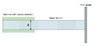# Estimation and validation of water pressure at nozzle

• Tomtom123
In summary, Chestermiller's experimental data shows that the pressure at the very center of the jet striking the wall is the same as the stagnation pressure, however the axial flow velocity is not stopped completely over an area equal to the jet coming out of the nozzle. The pressure from the jet hitting the wall is distributed over a much larger area, and the way to get the force that the jet exerts on the wall is to perform a momentum balance on the jet.

#### Tomtom123

ρ

I am trying to estimate and validate the pressure of water exiting a nozzle. For an unknown reason, the validation is consistently twice as high as the estimation.

Here the approach:
Estimation:
I am using the dynamic pressure equation for the estimation:
q = 1/2 * ρ * u2

where,
q = dynamic pressure
ρ = density of water (997 kg/m3)
u = liquid velocity
The velocity is calculated based on the known nozzle diameter and the flow rate (u = flow rate / nozzle area)

Validation:
The measurement is performed by simply measuring the jet force with a force gauge in horizontal orientation and in short distance (<1cm) - see the schematic. The assumption is that the jet diameter is the same as the nozzle.
q_val = jet force / nozzle area.

As mentioned before, in varying measurements the validation is close to being twice of the estimation data.
Is there anything I'm missing here?

#### Attachments

•Setup.JPG
17.9 KB · Views: 445
You are not approaching this correctly. Your experimental result is exactly what would be expected. Are you familiar with the Macroscopic Momentum Balance equation?

Hi Chestermiller,

Thanks

Tomtom123 said:
Hi Chestermiller,

Thanks
You probably have the correct answer for the pressure only at the very center of the jet striking the wall. But the axial flow velocity is not stopped completely over an area equal to the jet coming out of the nozzle. The pressure from the jet hitting the wall is distributed over a much larger area. The way to get the force that the jet exerts on the wall is to perform a momentum balance on the jet. For this system, the macroscopic momentum balance on the jet in the axial direction reduces to $$F=0-\dot{m}v$$where F is the axial force that the wall exerts on the fluid in the positive x direction, ##\dot{m}=\rho v A## is the mass flow rate of the jet, A is its cross section area, and the right hand side of the equation represents the rate of change of axial momentum of the jet. So the force that the wall exerts on the jet is: $$F=-\rho v^2A$$. And the force that the jet exerts on the wall is ##+\rho v^2A##. This is twice your stagnation flow estimate, but is consistent with your observed value of the force.

## 1. How is water pressure at a nozzle estimated?

The water pressure at a nozzle can be estimated by using a pressure gauge or by measuring the velocity of water at the nozzle outlet using a Pitot tube. Other factors such as the flow rate, nozzle size, and distance from the source can also affect the estimation of water pressure.

## 2. Why is it important to validate the estimated water pressure at a nozzle?

Validating the estimated water pressure at a nozzle is important to ensure the accuracy of the measurement. It also helps in identifying any discrepancies or errors in the estimation process, which can affect the performance of the nozzle and the overall system.

## 3. What methods are used for validating water pressure at a nozzle?

There are various methods for validating water pressure at a nozzle, including conducting a pressure test using a calibrated pressure gauge, using a fluid dynamics simulation software, or comparing the estimated pressure to the manufacturer's specifications for the nozzle.

## 4. Can the estimation and validation of water pressure at a nozzle vary for different types of nozzles?

Yes, the estimation and validation of water pressure at a nozzle can vary for different types of nozzles. This is because different types of nozzles have different designs and flow characteristics, which can affect the water pressure at the nozzle outlet.

## 5. How often should the estimation and validation of water pressure at a nozzle be conducted?

The estimation and validation of water pressure at a nozzle should be conducted regularly, especially if the nozzle is used frequently or if there are any changes in the system. It is recommended to conduct these tests at least once a year to ensure the proper functioning and safety of the nozzle.